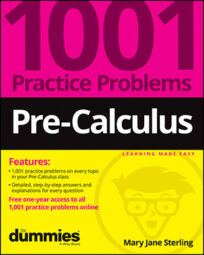##### Pre-Calculus: 1001 Practice Problems For Dummies (+ Free Online Practice)A sequence is a list of items; in mathematics, a sequence usually consists of numbers such as 1, 2, 4, 8, . . . or 1, 1, 2, 3, 5, 8, 13, . . . See the patterns in these two sequences? You need to know how to find patterns in lists of numbers so you can write the rest of the numbers in the list.

You can classify many sequences by type, which helps you determine particular terms or sums. Recognize the type, and you’re pretty much home free when doing your calculations.

You’ll work on sequences and series in the following ways:

• Finding a particular term in a sequence when given the general formula

• Determining a formula or standard expression for all the terms in a sequence

• Writing terms in a recursively formed sequence

Don’t let common mistakes trip you up; keep in mind that when working with sequences, some challenges will include

• Correctly formulating terms in a sequence by using the order of operations

• Recognizing when a sequence is arithmetic or geometric

• Assigning the correct signs in the terms of an alternating sequence

## Practice problems

1. Given the general term of the sequence, find the fifth term: an = 2n – 1

You find the fifth term, a5, by replacing the n with 5 in the general term and simplifying:

2. Write an expression for the general term of the sequence: 1, 2, 6, 24, 120, . . .